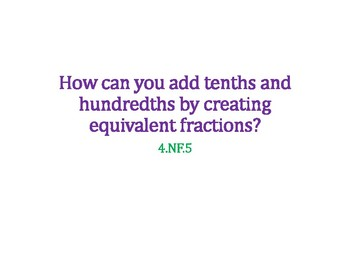# Add Tenths and Hundredths PowerPointSubject
Resource Type
File Type

PDF

(261 KB|14 pages)
Product Description
The PowerPoints typically include: Tiles/Links to Interactive Videos, Problem of the Day, Guided Practice, Independent Practice, and Agree or Disagree problems.

Purchase any of the other PowerPoints for Unit 3 separately or from the Unit 3 PowerPoint bundle in my store!

Unit 3 Fractions
-Lesson 1 Record tenths and hundredths as fractions and decimals
-Lesson 2 Add tenths and hundredths
-Lesson 3 How to add fractions with unlike denominators
-Lesson 4 How to subtract fractions with unlike denominators
-Lesson 5 Estimate sums and differences of fractions
-Lesson 7 Subtracting mixed numbers
-Lesson 8 Subtract with renaming fractions
-Lesson 9 Multiply a fraction by a whole number
-Lesson 10 Multiply a fraction by a fraction using a visual model
-Lesson 11 Multiply a fraction by a fraction without using a visual model
-Lesson 12 Multiply a whole number by a mixed number
-Lesson 13 Multiply a mixed number by a fraction
-Lesson 14 Multiply mixed numbers
-Lesson 15 Compare the size of the product to the factors
-Lesson 16 Represent division expressions as fractions
-Lesson 17 Divide a whole number by a unit fraction
-Lesson 18 Divide a fraction by a whole number
Total Pages
14 pages
N/A
Teaching Duration
N/A
Report this Resource to TpT
Reported resources will be reviewed by our team. Report this resource to let us know if this resource violates TpT’s content guidelines.